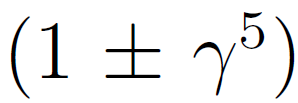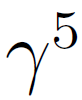# Funding Areas

FOR MORE RESEARCH TOPICS

## A Problem with the Electroweak Theory

Consider the following contradiction in the electroweak theory of the Standard Model.

1. The electroweak theory of neutrino interaction uses factors likein order to account for a complete parity violation. This factor implies a massless neutrino : “Nature had the choice of an aesthetically satisfying, but a left-right, symmetry violating theory, with a neutrino which travels exactly with the same velocity of light; or alternatively a theory where left-right symmetry is preserved, but the neutrino has a tiny mass – some ten thousand times smaller than the mass of the electron.”

The neutrino masslessness is also stated by other authors. A review article on neutrino properties states that “two-components left-handed massless neutrino fields play a crucial role in the determination of the charged current structure of the Standard Model” (see the Abstract of ). Similarly, a Quantum Field Theory textbook states: “Thus, massless neutrinos are a prediction of the Standard Model” (see , p. 555). Indeed, a massless neutrino is the basis for the two-component Weyl neutrino, which shows parity violation (see e.g. section 2.2 of ). The same argument appears on p. 139 of .

1. On the other hand, a recent review article negates the foregoing ides and states that it is now admitted “that neutrinos can no longer be considered as massless particles” (see , p. 1307). This statement is adopted by the Particle Data Group , which is the authorized organization for the definition of reliable particle data. The recognition of this fact by the community was demonstrated by the 2015 Nobel Prize, awarded to the persons who have discovered this property .

It follows that the experimentally confirmed massive neutrino undermines the basis of the Standard Model electroweak theory, since the massless neutrino is a crucial element in this theory.

Research topic: Can the validity of the electroweak theory be restored?

Remark: Furthercontradictions are discussed in .

#### References

 A. Salam, Nobel lecture, 8 December, 1979.

 S. M. Bilenky, Phys. Part. Nuclei 46, 475 (2015).

 D. Griffiths, Introduction to Elementary Particles, 2nd edition,Weinheim, Wiley-VCH, 2008.

 M. Srednicki, Quantum Field Theory (University Press, Cambridge, 2007).

 J. A. Formaggio and G. P. Zeller, Rev. Mod. Phys. 84, 1307 (2012).

 http://pdg.lbl.gov/2018/reviews/rpp2018-rev-intro-neutrino-prop.pdf

 https://www.nobelprize.org/prizes/physics/2015/summary/

 https://www.tau.ac.il/~elicomay/Chirality_1.pdf